# How does the binomial theorem work

What is that pasccal triangle?

 ...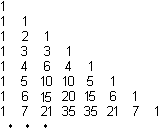... ...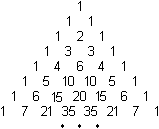... Pascal's triangle is an arrangement of numbers in a triangular shape,constructed according to a simple law of formation.

construction Top

 11   1 ...... The law of education is as follows.A triangle made up of three ones is assumed.The following lines also start and end with a one.In between there are numbers that result as the sum of the two numbers above.In this way, the triangle can be continued as far as desired.

Binomial coefficient Top
The numbers of the Pascal triangle thus emerge successively from each other.

..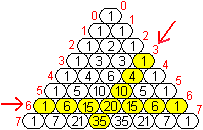....But there is also the option of calculating them independently of one another as so-called binomial coefficients.
So you get the number 20 in the horizontal 6th row and in a sloping 3rd column as follows.

 .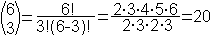.. One reads the bracket symbol as "6 over 3".On this page the term is also called C (6,3).

 In general, the number in the nth row and the kth column becomes according to the formula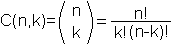calculated.
The formula goes back to Euler. It was found in a completely different context.
It indicates the number of different ways in which k objects can be selected from a set of n different objects without considering the order and without replacing.
This number is of interest, for example, when playing the lottery, where the aim is to find "6 correct" numbers from the first 49 numbers.
More on my page 13 983 816.

The term C (n, k) makes it possible to understand the construction principle C (n, k-1) + C (n, k) = C (n + 1, k) of Pascal's triangle.
Each number is the sum of the two numbers above it.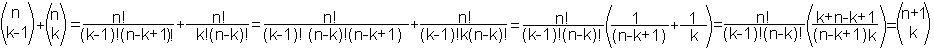For the sake of completeness, the edges of the triangle must be defined as C (0,0) = C (n, 0) = C (n, n) = 1.

The symmetry of Pascal's triangle results from the identity C (n.k) = C (n, n-k), as can be easily calculated.

Binomial theorem Top
The binomial theorem is about converting the power of a two-part sum into a sum.
The simplest case is the binomial formula (a + b) ² = a² + 2ab + b².
For the powers (a + b)n results for n = 2, ..., 7.

 (a + b)2 = (a + b)3 = (a + b)4 = (a + b)5 = (a + b)6 = (a + b)7 = a2+ 2ab + b2a3+ 3a2b + 3ab2+ b3a4+ 4a3b + 6a2b2+ 4ab3+ b4a5+ 5a4b + 10a3b2+ 10a2b3+ 5ab4+ b5a6+ 6a5b + 15a4b2+ 20a3b3+ 15a2b4+ 6ab5+ b6a7+ 7a6b + 21a5b2+ 35a4b3+ 35a3b4+ 21a2b5+ 7ab6+ b7

Lo and behold, the prefixes form the Pascal triangle if the summands are cleverly arranged.

In general: (a + b)n= C (n, 0) anb0+ C (n, 1) an-1b1+ C (n, 2) an-2b2+ ... + C (n, n-2) a2bn-2+ C (n, n-1) a1bn-1+ C (n, n) a0bn.
The method of complete induction is used to prove this formula.
That is on the English language Wikipedia page Binomial theorem (URL below) demonstrated.

The name binomial coefficient for C (n, k) introduced above finds an explanation here.

Special case
 ...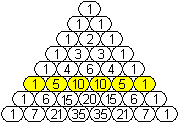... If one sets a = b = 1, then 2n is equal to the sum of the numbers in the nth row.1+5+10+10+5+1 = 25C (n, 0) + C (n, 1) + C (n, 2) + ... + C (n, n-2) + C (n, n-1) + C (n, n) = 2n

Pascal numbers Top
In this section some statements from an article from "Bild der Wissenschaft" from 1965 are reproduced (1).
Apparently the author did not use the computer at the time.

definition
 ...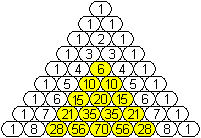... If you leave out the ones at the edge of Pascal's triangle and the natural numbers in the first columns, the Pascal's numbers remain.The first numbers are 6, 10, 15, 20, 21, 28, 35, 36, 45, 55, 56, 66, 70, 78, 84, 91, 105, 120, 126, 136, 153, 165, 171, 190, 210, 220, 231, 252, 253, 276, 286, 300, 325, 330, 351, 364, 378, 406, 435, 455, 462, 465, 495, 496, 528, 560, 561, 595, 630, 666, 680, 703, 715, 741, 780, 792, 816, 820, ...

Number of Pascals numbers up to the 100th line
 ...... First of all, a restriction to the first eight Lines.The number of numbers is determined by the following consideration.> The number of marked numbers is 1 + 2 + 3 + 4 + (8-3) = (5 * 6): 2 = 15.> (8-2): 2 = 3 numbers in the vertical symmetry axis occur once.> 15-3 = 12 numbers appear twice. That leads to 12: 2 = 6 numbers.So in total there are 6 + 3 = 9 numbers.
This number could of course be obtained directly by counting. But so one can generalize.

The number of numbers in the first 100 lines is obtained by replacing the number 8 with 100.
> The number of marked numbers is 1 + 2 + ... + (100-3) = (97 * 98): 2 = 4753.
> (100-2): 2 = 49 numbers occur once along the vertical axis of symmetry.
> 4753-49 = 4704 numbers appear twice. That leads to 4704: 2 = 2352 numbers.
So there are a total of 2352 + 49 = 2401 numbers.

This number has to be reduced, because there are other identical numbers in the triangle that are not in one line.
 C (16.2) = C (10.3)=120 C (21.2) = C (10.4)=210 C (56.2) = C (22.3)=1540 C (78.2) = C (15.5)= C (14.6)=3003 C (120.2) = C (36.3)=7140 C (153.2) = C (19.5)=11628 C (221.2) = C (17.8)=24310

Distribution of Pascal's numbers
According to (1) there is
 a single digit number (the six) 15 two-digit numbers 48 three-digit numbers 135 four-digit numbers 393 five-digit numbers 1140 six-digit numbers 3398 seven-digit numbers .
So among the first 10,000,000 numbers there are only 1 + 15 + 48 + 135 + 393 + 1140 + 3398 = 5130 Pascal numbers.
That's just 5130: 10,000,000 = 0.000513% of all numbers.

Pattern in Pascal's triangle Top
Because of the factorials in C (n, k) = n! / [K! (N-k)!], Pascal's numbers are rich in factors.
In (1) the typical number C (27.8) = 2,220,075 = 33*52* 11 * 13 * 23 specified.

Apparently the distribution has the divider system. It is noteworthy that triangles on the tip are created when numbers are marked with equal divisors. Here are the patterns for easy dividers.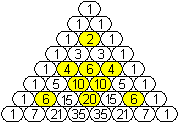divisible by 2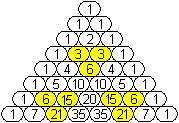divisible by 3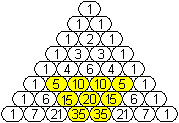divisible by 5
The patterns become more impressive when you look at more lines. I refer to the applets by Arndt Brünner and shodor.org (URL below).

Worth seeing: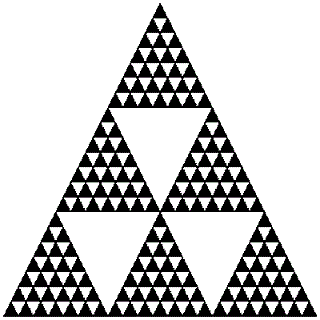divisible by 7

Consequences in Pascal's triangle Top
Triangular numbers

 ...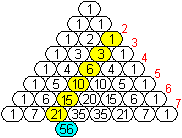...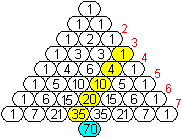... In the columns there are sequences, namely > in the 0th column the stagnant sequence of ones, > in the 1st column the sequence of natural numbers, > in the 2nd column the sequence of triangular numbers, > in the 3rd column the sequence of the tetrahedral numbers, > in the 4th column the sequence of numbers for the 4th dimensional tetrahedron, > in the 5th column the sequence of numbers for the 5th dimensional tetrahedron etc.
There is a connection between the consequences.
Every sequence to the right of a sequence is always the sequence of the partial sums of the previous one.
E.g. the triangle sequence 1, 3, 6, 10, 15, ... is also the sum sequence 1, 1 + 2, 1 + 2 + 3, 1 + 2 + 3 + 4, 1 + 2 + 3 + 4 + 5, ....
This explains why in the above drawing the sum of the numbers in the yellow fields is equal to the number in the blue field.

Catalan numbers
The Catalan numbers indicate into how many triangles an n-gon is divided by the diagonals.
The first parts of the sequence are 1, 2, 5, 14, 42, 132, 429, 1430, 4862, 16796, ... (Sloane's A000108).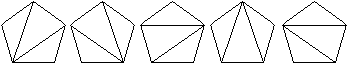The Catalan number 5 belongs to the pentagon.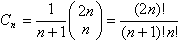Education Act

 ...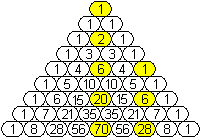... The sequence of Catalan numbers can be read off in Pascal's triangle by calculating the difference between the number on the axis of symmetry and the next but one number in a line.These are 1, 2, 6-1, 20-6, 70-28, ...

Fibonacci sequence
The Fibonacci sequence results when each term in the sequence is calculated as the sum of the two preceding terms.
The starting point is the first two terms 1.1. This leads to 1, 1, 2, 3, 5, 8, 13, 21, 34, 55, ...
(This is reminiscent of the construction of Pascal's triangle above.)
 ...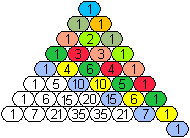... The terms of the sequence are contained in Pascal's triangle from the 3rd term on as sums.These are the sums of diagonal numbers.1+1=2, 2+1=3, 1+3+1=5, 3+4+1=8, 1+6+5+1=13, 4+10+6+1=21, 1+10+15+7+1=34, ...

Harmonic triangle Top

 ...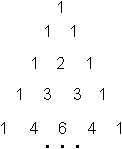... The harmonic triangle or Leibniz triangle emerges from the Pascal triangle.

 ...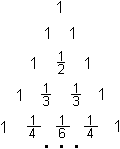The first step is to form the reciprocal values ​​of the numbers.This means that every number z is replaced by 1 / z.

 ...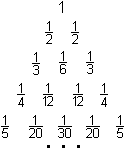... In a second step you divide the numbers of each line by the number of the line increased by 1,i.e., the number in the zeroth line by 1, that in the first line by 2, that in the second line by 3, and so on.This is how the harmonious triangle is created.

The numbers C (n, k) of the Pascal triangle are thus replaced by 1 / [(n + 1) C (n, k)].

What is special is that in the harmonic triangle every number is the sum of the two underneath lying numbers is.
That means in the formula language 1 / [(n + 1) C (n, k)] = 1 / [(n + 2) C (n + 1, k)] + 1 / [(n + 2) C (n + 1, k + 1)].
Confirmation:
1 / [(n + 2) C (n + 1, k)] + 1 / [(n + 2) C (n + 1, k + 1)] =
[k! (n + 1-k)!] / [(n + 2) (n + 1)!] + [(k + 1)! (nk)!] / [(n + 2) (n + 1 )!] = [k! (nk)!] / [(n + 1) n!] {(n + 1-k) / (n + 2) + (k + 1) / (n + 2)} = [k! (nk)!] / [(n + 1) n!]
= 1 / [(n + 1) C (n, k)]

Pascal's triangle on the Internet Top

Arndt Brünner
Pattern in Pascal's triangle

Frederik Schäfer
Poission distribution, binomial distribution and hypergeometric distribution

Manfred Börgens (mathematics on postage stamps)
Blaise Pascal (1623 - 1662), Pascal's triangle with old Chinese decimal numbers

Matroids math planet
The Pascal triangle, the trinomial triangle

Michael Holzapfel
The Pascal triangle

Walter Fendt
Binomial coefficients and Pascal's triangle (applet)

Wikipedia
Pascal's triangle, binomial theorem, binomial series, binomial coefficient, Sierpinski triangle, Fibonacci sequence,
Catalan numbers, harmonic triangle

English

Eric W. Weisstein (MathWorld)
Pascal's Triangle, Pascal's Formula, Binomial Coefficient, Binomial Sums, Sierpinski Sieve, Tetrix,
Number triangle

Math forum
Pascal's Triangle: Binomial Coefficient, Probability and Combinations, Sierpinski Triangle

shodor.org
Coloring Multiples in Pascal's Triangle

The On-Line Encyclopedia of Integer Sequences
Binomial coefficients: C (n, k), 2 <= k <= n-2, sorted, duplicates removed. (A006987)
Numbers that occur 5 or more times in Pascal's triangle (A003015)

Wikipedia
Pascal's triangle, Pascal's rule, Binomial theorem, Binomial series, Binomial coefficient, Star of David theorem,
Sierpinski triangle, Fibonacci number, Catalan number, Leibniz harmonic triangle

credentials Top
(1) Siegfried Rösch: News from the Pascal Triangle, Bild der Wissenschaft, September 1965 (page 758ff.)
(2) Martin Gardner: Mathematical Carnival, Frankfurt am Main, 1975 [ISBN 3-550-07675-4]

Feedback: Email address on my main page

URL of my homepage:
http://www.mathematische-basteleien.de/